# Excel: vlookup vs. index & match

In this blog, I presented 3 excel functions, vlookup, index, and match, on their usages, syntaxes, applied them in different use cases, and their pro & cons.

During my work, sometimes I need to create a dynamic Excel workbook for clients. The screenshot below is a simple example, we can filter “analysed year”, “compared year” and “country” to get some KPIs of selected country on selected year.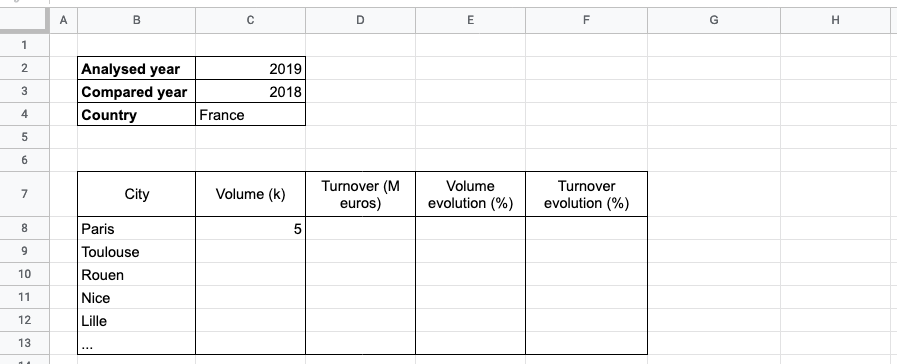How can we look for a value with multiple conditions? In this blog, I’ll show you how to accomplish it with functions vlookup or index & match with following points:

• Usage
• Example
• Pro & cons

## VLOOKUP function

### Usage

Use VLOOKUP when you need to find things in a table or a range by row. For example, look up the price of an automotive part by the part number, or find an employee name based on their employee ID.

Syntax

VLOOKUP (lookup_value, table_array, col_index_num, [range_lookup])

• lookup_value: (required) The value you want to look up. The value you want to look up must be in the first column of the range of cells you specify in the table_array argument.
• table_array: (required) The range of cells in which the VLOOKUP will search for the lookup_value and the return value.
• col_index_num: (required) The column number (starting with 1 for the left-most column of table_array) that contains the return value.
• range_lookup: (optional) A logical value that specifies whether you want VLOOKUP to find an approximate or an exact match: Approximate match - 1/TRUE, Exact match - 0/FALSE.

### Example

Now back to the use case at the beginning of the blog, how can we get Paris’ “volume(k)” in 2019 vs. 2018 with the following worksheet?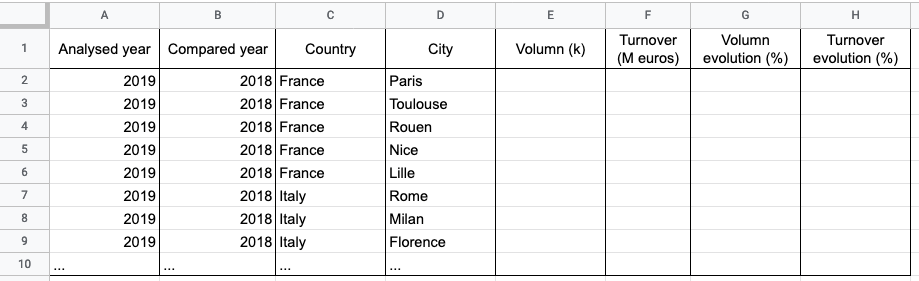Considering there are multiple values to look up, and the lookup_value can only be one column, so what we can do is concatenating columns “Analysed year”, “Compared year”, “Country” and “City” into a new column named “_param” with CONCATENATE function.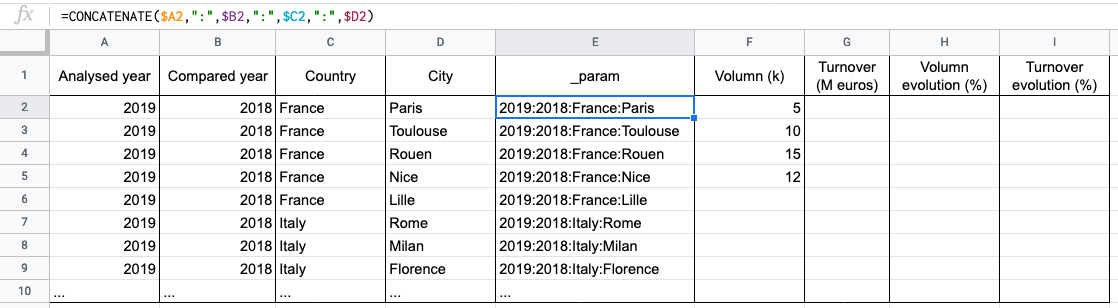Then we can get the result with vlookup as following: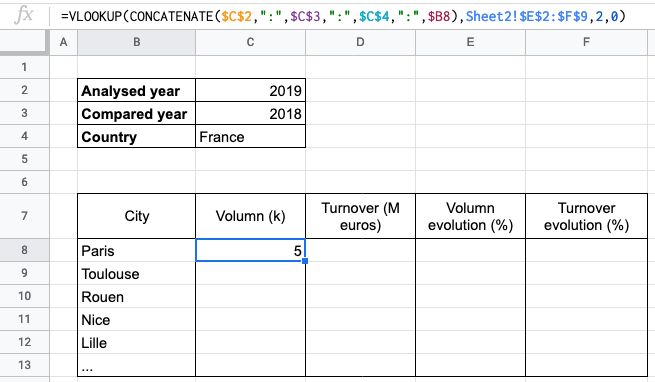### Pro & cons

vlookup is efficacy and easy to use, since it looks for value only in one column and search its result in one column as well, but if you want to look for multiple values like the example, you need to concatenate them as the “lookup_value” firstly, then apply the vlookup function.

## INDEX + MATCH function

### Usage

INDEX function
The INDEX function returns a value or the reference to a value from within a table or range. Here I will mainly talk about the case when you want to return the value of a specified cell or array of cells (so-called Array form), if you want to return a reference to specified cells, see Reference form.

Returns the value of an element in a table or an array, selected by the row and column number indexes. Use the array form if the first argument to INDEX is an array constant.

Syntax

INDEX(array, row_num, [column_num])

• array: (required) A range of cells or an array constant.
• If the array contains only one row or column, the corresponding row_num or column_num argument is optional.
• If the array has more than one row and more than one column, and only row_num or column_num is used, INDEX returns an array of the entire row or column in the array.
• row_num: (required) Unless column_num is present. Selects the row in the array from which to return a value. If row_num is omitted, column_num is required.
• column_num: (optional) Selects the column in the array from which to return a value. If column_num is omitted, row_num is required.

MATCH function
The MATCH function searches for a specified item in a range of cells, and then returns the relative position of that item in the range. For example, if the range A1:A3 contains the values 5, 25, and 38, then the formula =MATCH(25,A1:A3,0) returns the number 2, because 25 is the second item in the range.

Syntax

MATCH(lookup_value, lookup_array, [match_type])

• lookup_value: (required) The value that you want to match in lookup_array. The lookup_value argument can be a value (number, text, or logical value) or a cell reference to a number, text, or logical value.
• lookup_array: (required) The range of cells being searched.
• match_type: (optional) The number -1, 0, or 1. The match_type argument specifies how Excel matches lookup_value with values in lookup_array. The default value for this argument is 1.
• 1 or omitted: MATCH finds the largest value that is less than or equal to lookup_value.
• 0: MATCH finds the first value that is exactly equal to lookup_value.
• -1: MATCH finds the smallest value that is greater than or equal to lookup_value.

### Example

Same as above, let’s back to the use case at the beginning of the blog, how can we get Paris’ “volume(k)” in 2019 vs. 2018 with the following worksheet?Then we can get the result with index & match as following: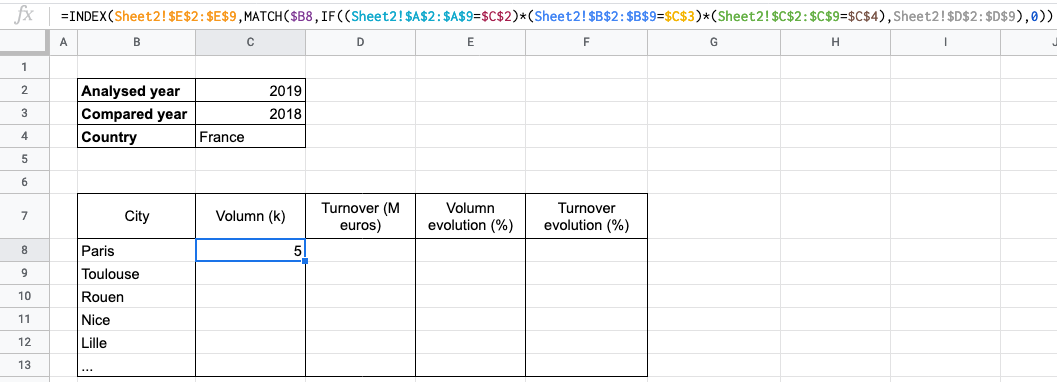We can use INDEX to help us get the result, so we set the volume’s column of sheet2 as array, set “0” as column_num, it remains row_num to set. In order to find out the row_num, we need the help of MATCH. First, we set one of “Analysed year”, “Compared year”, “Country” and “City” as the lookup_value, here I take “City”, then set the ranges which is equal to each other conditions as lookup_array, and find the first value that is exactly equal to lookup_value.

### Pro & cons

Inverse to the negative points of vlookup, we don’t need to concatenate all conditions into one column, we can intersect different conditions as many as you want with MATCH, but the cost is it might take a long time to find the final result.

## Conclusion

In this blog, I presented 3 excel functions, vlookup, index, and match, on their usages, syntaxes, applied them in different use cases, and their pro & cons. Hope it’s useful for you!